How to Use the CEILING Excel Formula: Functions, Examples and Writing Steps - Compute Expert

# How to Use the CEILING Excel Formula: Functions, Examples and Writing Steps

Home >> Excel Tutorials from Compute Expert >> Excel Formulas List >> How to Use the CEILING Excel Formula: Functions, Examples and Writing Steps

In this tutorial, you will learn how to use the CEILING excel formula completely.

When working with numbers in excel, we sometimes need to round up a number to a certain multiple. If we use CEILING to do the rounding process, then we can get the result easily!

Want to master the method to use this CEILING formula in excel? Read this tutorial until its last part!

Disclaimer: This post may contain affiliate links from which we earn commission from qualifying purchases/actions at no additional cost for you. Learn more

## What is the CEILING Formula in Excel?

CEILING formula in excel is a formula that can help us in a number rounding up process to a particular multiple.

## CEILING Function in Excel

We can use CEILING to round up a number to a multiple that we determine.

## CEILING Result

We will get a number which is the result of our number rounding up process.

## Excel Version from Which We Can Use CEILING

We can use CEILING since excel 2003.

## The Way to Write It and Its Inputs

Here is the general writing form of a CEILING formula in excel.

= CEILING ( number_to_round , multiple )

And here is the explanation of the inputs we need to give in its formula writing.
• number_to_round = the number we want to round up to a certain multiple
• multiple = the multiple which we round up our number to

## Example of Its Usage and Result

Here is the usage and result example of the CEILING formula in excel.As you can see there, CEILING will always round up the number we input into it to the multiple we want. When we input negative numbers, CEILING will round our number away from zero.

## Writing Steps

After discussing the writing form, inputs, and implementation example of CEILING, now let’s discuss its writing steps. As the inputs of this formula are quite simple, you should be able to understand the writing steps easily!

1. Type an equal sign ( = ) in the cell where you want to put your CEILING result2. Type CEILING (can be with large and small letters) and an open bracket sign after =3. Input the number you want to round up to a certain multiple. Then, type a comma sign ( , )4. Input the multiple you want to round up your number to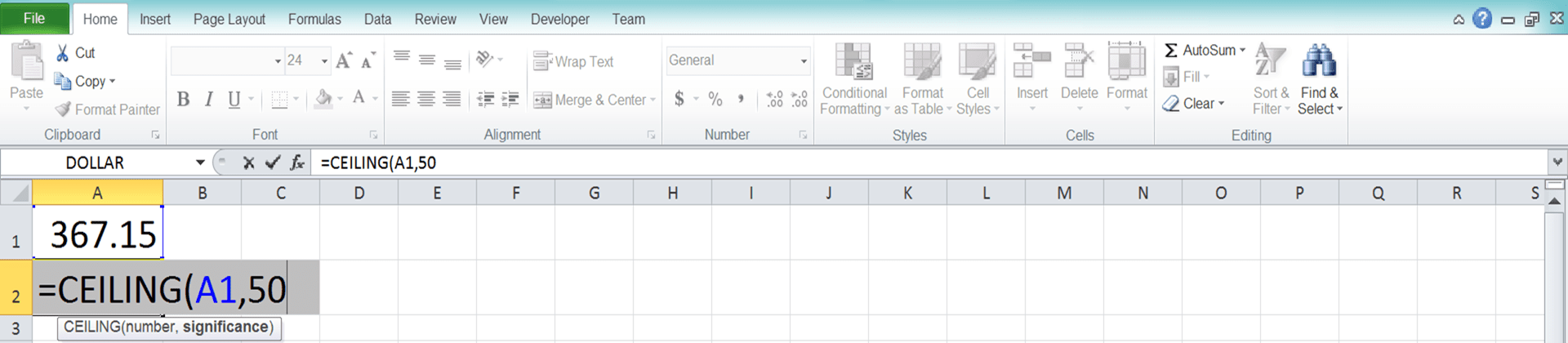5. Type a close bracket sign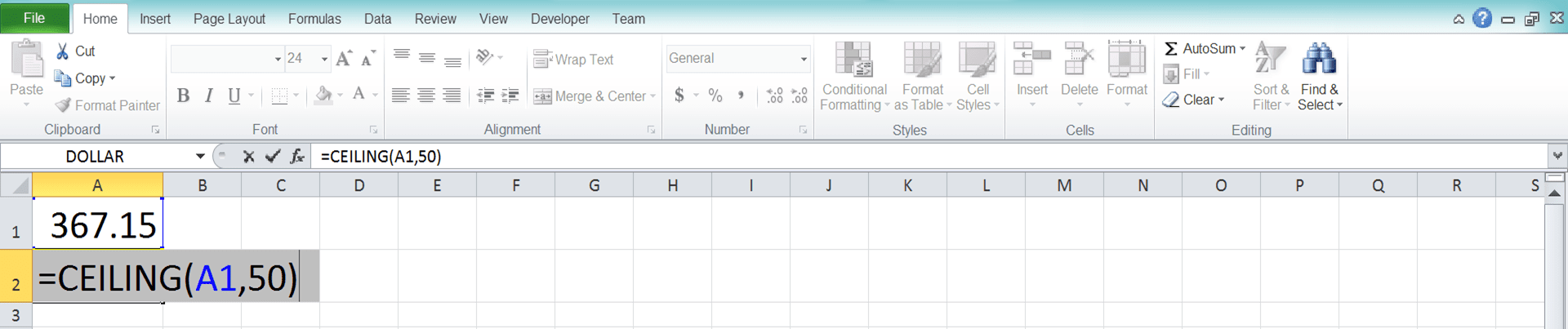6. Press Enter
7. Done!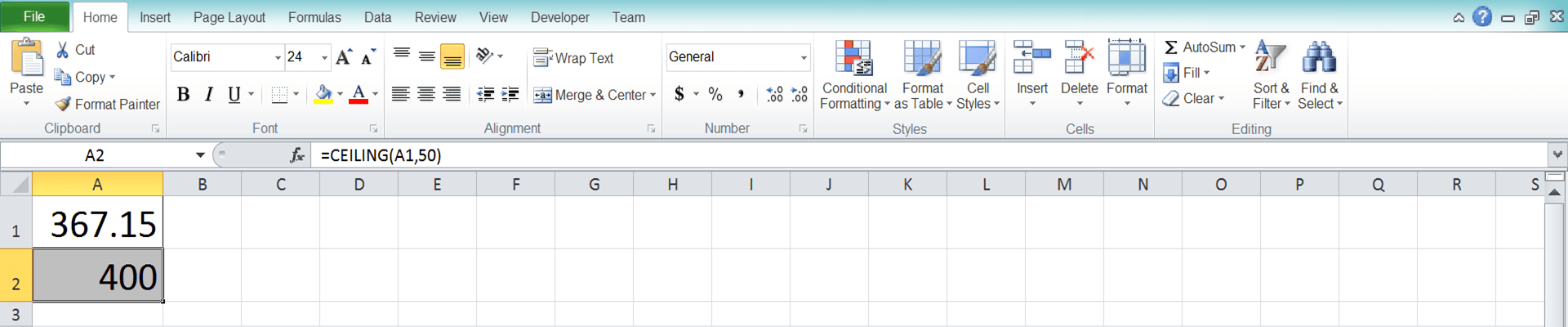## CEILING to Round Up Date

As the date is also a number data, we can use CEILING to round it up to a certain days multiple. Just input the date we want to round up and the days multiple we want to round our date to.

Here is the CEILING general writing form in excel to round up a date.

= CEILING ( date_to_round , days_multiple )

And here is its implementation example.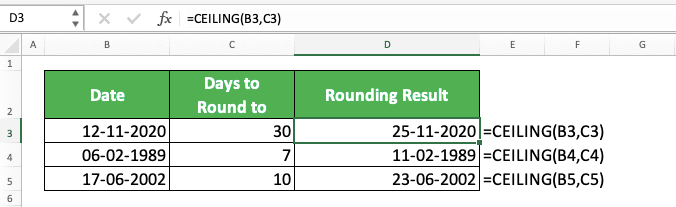If you get a number from CEILING when you round up your date, just change its data type to date.

## CEILING to Round Up Time

As time is a number data too, we can also round up time data using CEILING. Just input the time data you want to round up and the time multiple you want to round it to.

The time multiple you input to CEILING to round up time can be in hours, minutes, or seconds. Input it in the form of time data too so you can get your result correctly.

Here is the CEILING general writing form in excel to round up time.

= CEILING ( time_to_round , time_multiple )

And here is its implementation example.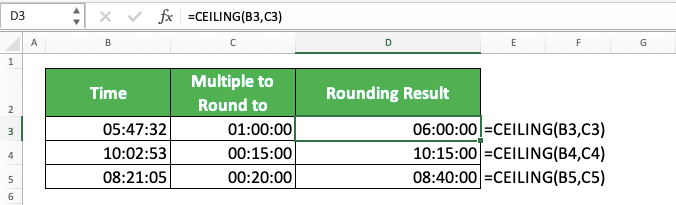Similar to date, if you get a number from CEILING instead of time, you should change the data type to time.

## CEILING Alternative: CEILING.MATH

Since excel 2013, you can use CEILING.MATH as an alternative to CEILING.

If we look at the basic function, CEILING.MATH is the same as CEILING. They both help you to round up your number to a certain multiple.

There are two things, however, that become crucial differences when it comes to CEILING and CEILING.MATH.
• CEILING doesn’t have a default multiple input. That means you have to input a multiple to CEILING for the formula to run.

CEILING.MATH, meanwhile, has a default multiple input of 1. If you don’t give any multiple input to CEILING.MATH, it will round up your number to the multiple of 1

• You can control your negative number rounding process with CEILING.MATH while you can’t do that with CEILING. If you use CEILING, you will round your negative number away from zero.

If you use CEILING.MATH, however, you can input whether you want to round it closer to or away from zero

Here is the general writing form of CEILING.MATH in excel.

= CEILING.MATH ( number_to_round , [ multiple ] , [ negative_number_rounding_mode ] )

You have one required input and two optional inputs in CEILING.MATH. For the required input, you must input the number you want to round as the first input of your CEILING.MATH.

For the negative number rounding mode, the default is you round your negative number closer to zero. If you input any non-zero value for it, you will round your negative number away from zero. If you input zero or don’t input anything, you will round it closer to zero.

For a better understanding of the formula, here is the implementation example of CEILING.MATH in excel.As you can see in the example, CEILING and CEILING.MATH round up positive numbers the same way. When we don’t input a multiple to CEILING.MATH, it will round our number up to the multiple of 1.

When we round a negative number, however, the default nature of CEILING.MATH is to round it closer to zero (the opposite of CEILING). We can input a non-zero value as its negative number rounding mode to round our negative number away from zero (TRUE is the same as 1 in excel, hence the rounding result for the third number in the example).

When you need the differences that CEILING.MATH gives, use it in your formula writing!

## Exercise

After you have learned how to use the CEILING in excel, you can sharpen your understanding by doing the exercise below!

### Questions

1. What is the production quantity for the benchmark of the company’s factory if the standard batch is 10?
2. What is the production quantity for the benchmark of the company’s factory if the standard batch is 50?
3. What is the production quantity for the benchmark of the company’s factory if the standard batch is 1000?

If you round up a positive number to a negative multiple using CEILING, you will get a #NUM error.

Related tutorials you should learn from:

Get updated excel info from Compute Expert by registering your email. It's free!